# Mathematics 1010 online

A quadratic equation can be written in the standard form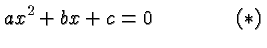where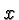is the unknown variable, the coefficients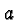,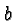and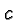are real numbers, and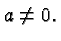(If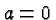we have a linear equation . and a much simpler problem.) In a class like this the coefficients are usually given explicitly, but in actual applications they are often variables, or even algebraic expressions.

To solve the quadratic equation means to find values ofthat make the equation true. To illustrate the principles and issues let's look at a special case first. Consider the equation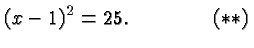You might say that's not a quadratic equation since it's not in the form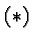. However, it can be converted into an equivalent equation that is in that form, by suitable operations on both sides of the equation: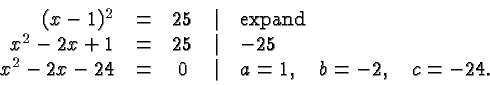The last equation in this sequence is in standard form, with,, andhaving the given values. However, the equation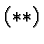can be solved much more easily than: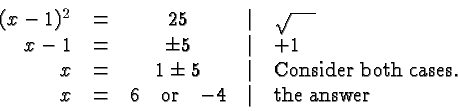Thus there are two solutions of the equation,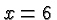and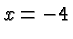. We can (and should) verify this by substituting these values in the original equation. Ifwe obtain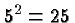and ifwe obtain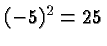.

Note the symbol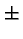in the second and third of the above sequence of equations. The square root of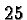is positive by convention and equals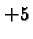. However, our task at that stage is not to compute a square root as such, but to answer the question for what values ofdoesequal? There are two such values,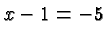and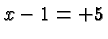, and we must consider both possibilities.

Let's now consider the more general equation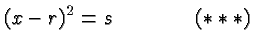where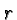and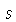are considered known and, as before,needs to be determined.

It can be solved just like the special case considered earlier: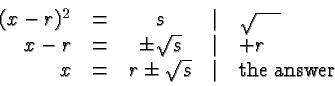Let's see how this works with our equation in standard form: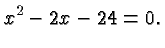If the constant term was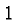instead of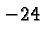we would have a perfect square . To make it so we just addon both sides and obtain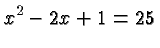which can be rewritten as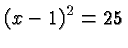Note that since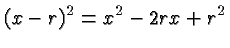we simply look at the factor of, halve it, square it, and add the appropriate constant that makes the constant equal to that desired value. One simple minded way to obtain that constant is to subtract whatever constant is there, and then add the desired value. For this to work the leading coefficient (multiplying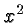) must equal. If it doesn't we divide first by the leading coefficient on both sides. (However, do not memorize this procedure as a recipe. Rather think about it, and practice it, until it makes sense and is so compelling that it becomes a natural part of your repertoire.)

### Two Real Solutions

An example illustrates the process: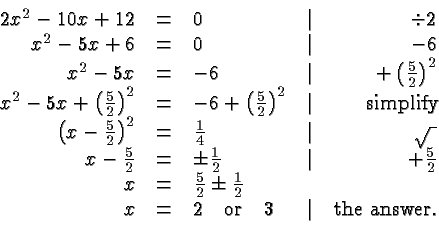We easily check that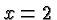and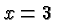do in fact satisfy the original equation.

The above example illustrates one of three possible outcomes of this procedure, the case where there are two real solutions.

### One Real Solution

In the following example there is only one solution: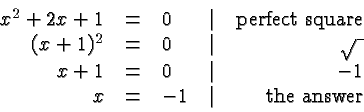The reason there is only one solution is the fact that there is one and only one number whose square is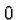.

### Two Conjugate Complex Solutions

If the above procedure leads to taking the square root of a negative number we obtain a conjugate complex pair of solutions as illustrated in the following example: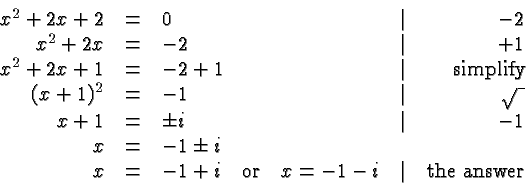Of course there is nothing to stop us from applying this procedure to the general equation. This gives rise to the quadratic formula . Personally, I prefer not to burden my mind with having to memorize reliably yet another formula, and so I complete the square almost every time I solve a quadratic equation.